There is no specific operator to write exponents in Java, but there are several other ways to do exponents in Java. You can do it by using a built-in method of static class named Math or by doing it by yourself using for loop or recursive call. The former method `pow()`takes two arguments, and the function will return the 1st parameter raised to the power of the 2nd parameter. By doing it using for loop or recursive call, the exponent must be greater than or equal to 1, and if the exponent is less than 1, then it could not be handled using later two methods. The detail of each method with examples and sample code is as following:

## 1. Find Exponents in Java Using the Built-in Method of ‘Math’ Library

The supported operations of this class include common trigonometric functions, finding absolute value, exponents, and rounding. To use this method, you have to follow these steps:

Step 1:

You have to first open any java IDE e.g., eclipse or net beans, then create a new class or write the code to the existing class. To create a new class, click on ‘File’ and then on ‘New Project.’Select ‘java’, then ‘java application’ and press the ‘Next’ button.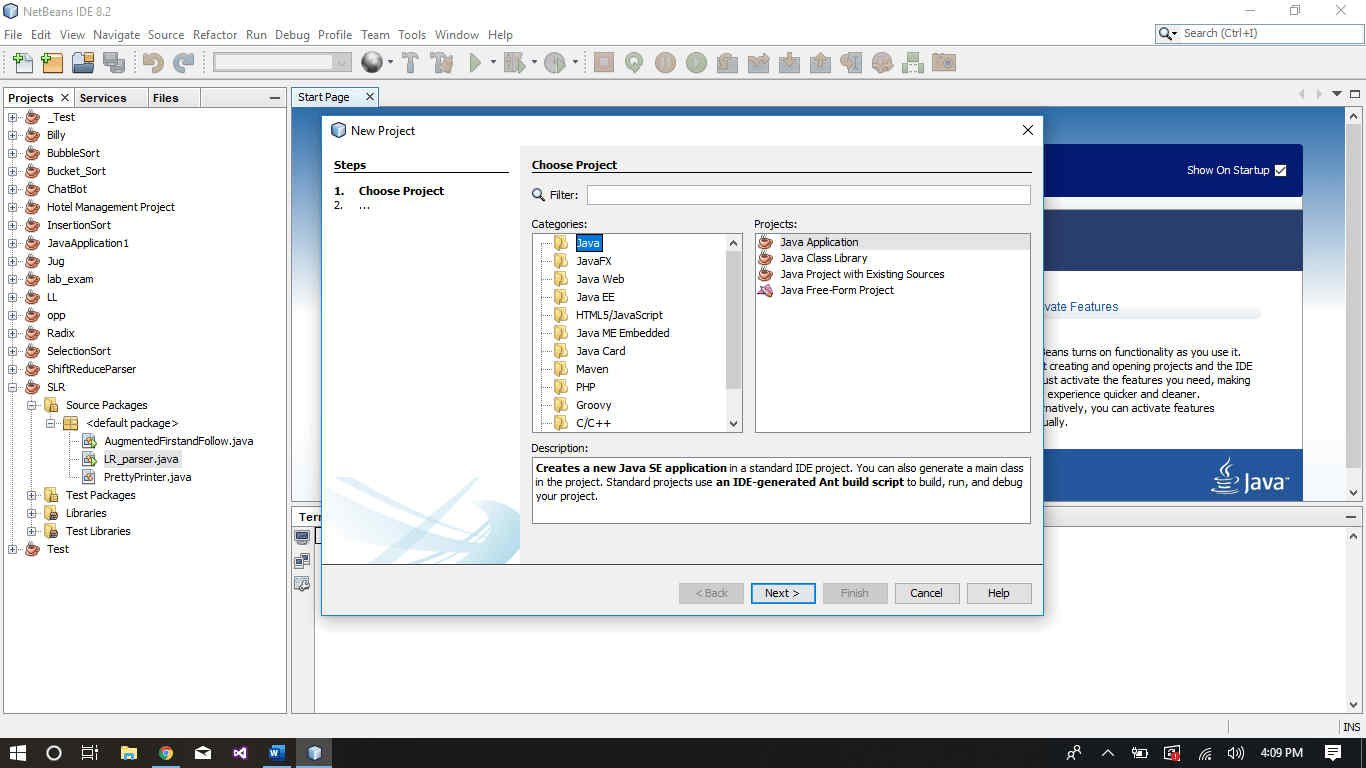Write the name of the class and then click on the ‘Next’ button.

Step 2:

Now you have to write the code, first, write the following line at the top of the class to import the header file

Related Posts
`import java.lang.Math;`

Write the following code anywhere you want to find the exponent.

`double answer = Math.pow(num, exp);`

where num is the number whose exponent you want to find and exp is the raised power(exponent to be found) of that number. Following code is an example of this method: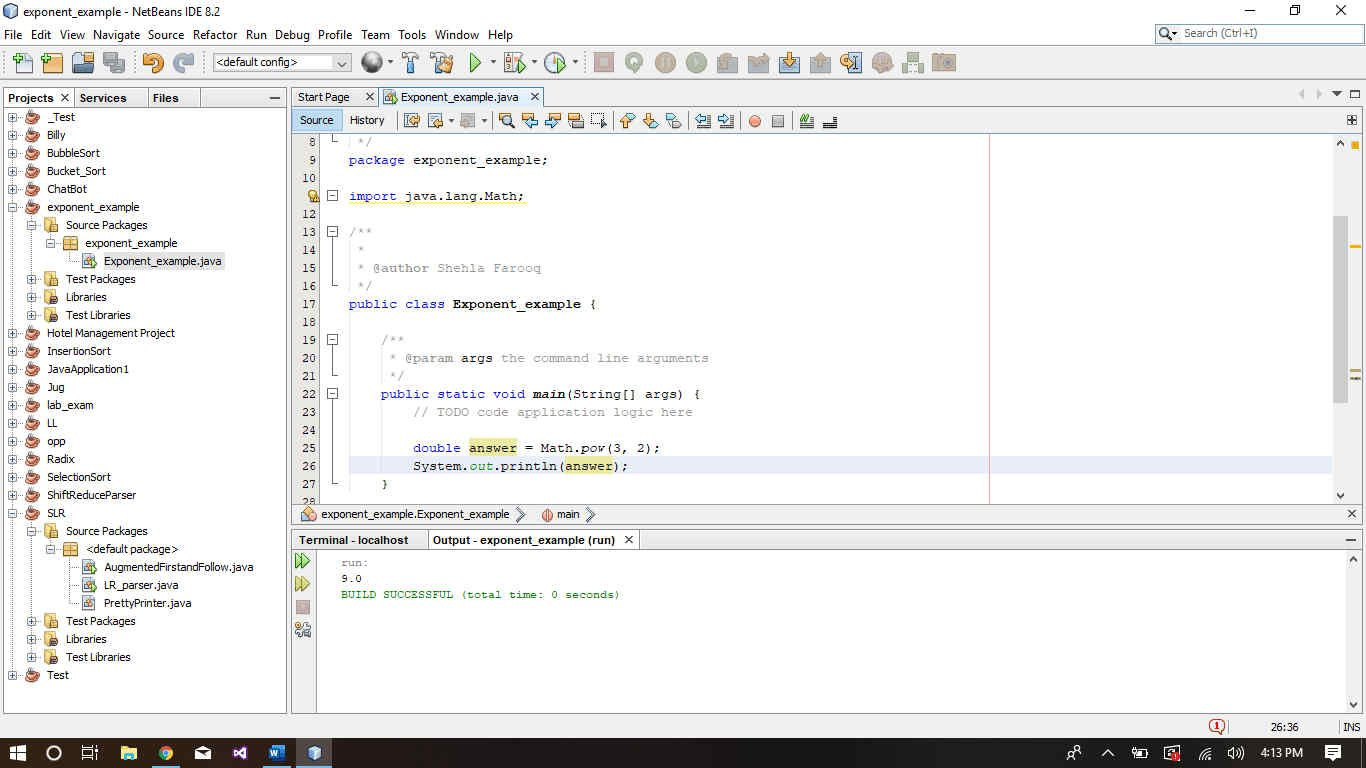The resulting answer will have the data type double, in order to change the data type, you have to convert the result manually.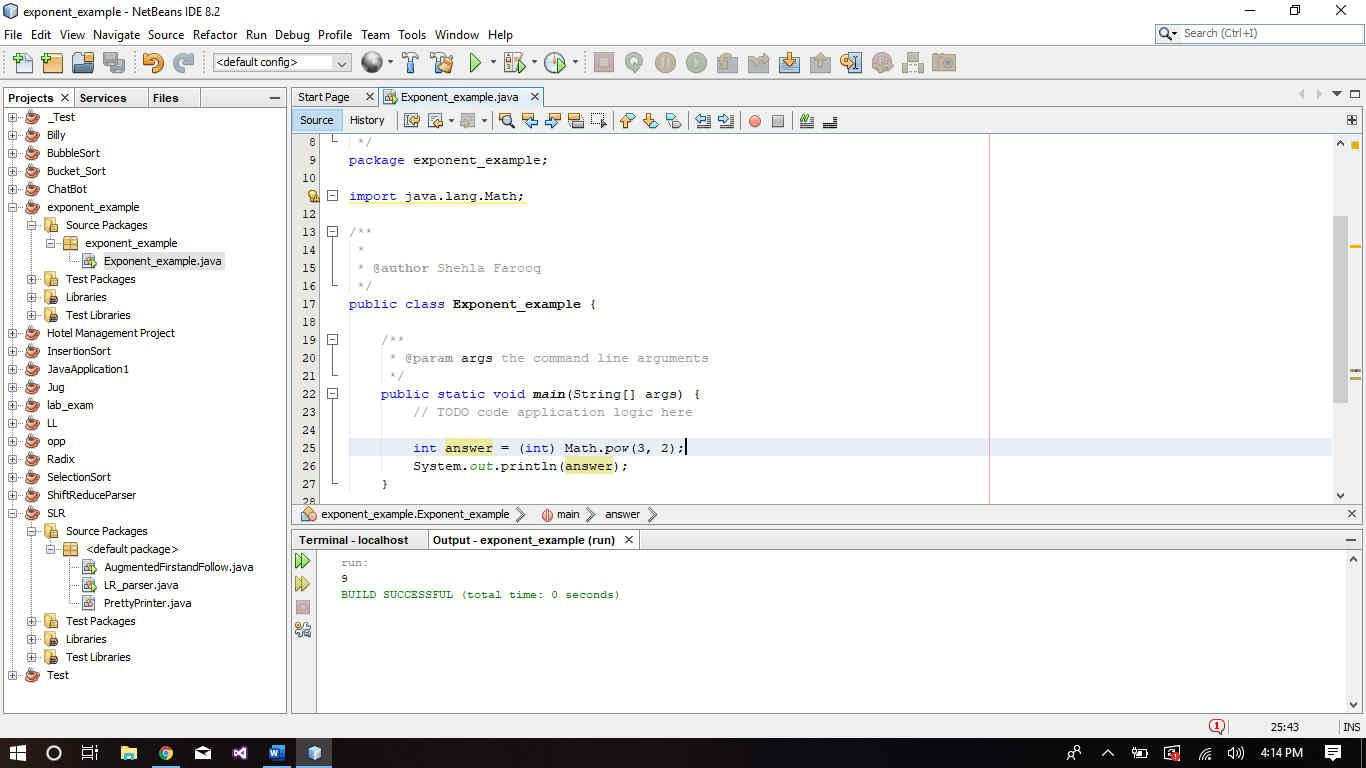## 2. How to do Exponents in Java Using for-loop

You can also do it by yourself by using a for loop. But this method is only valid for the exponent >= 1.

```package exponent_example;
public class Exponent_example {
public static void main(String[] args) {
double num = 3;
int exp = 2;
}
public static double Pow(double num, int exp){
double result =1;
for (int i = 0; i < exp; i++) {
result *= num;
}
return result;
}
```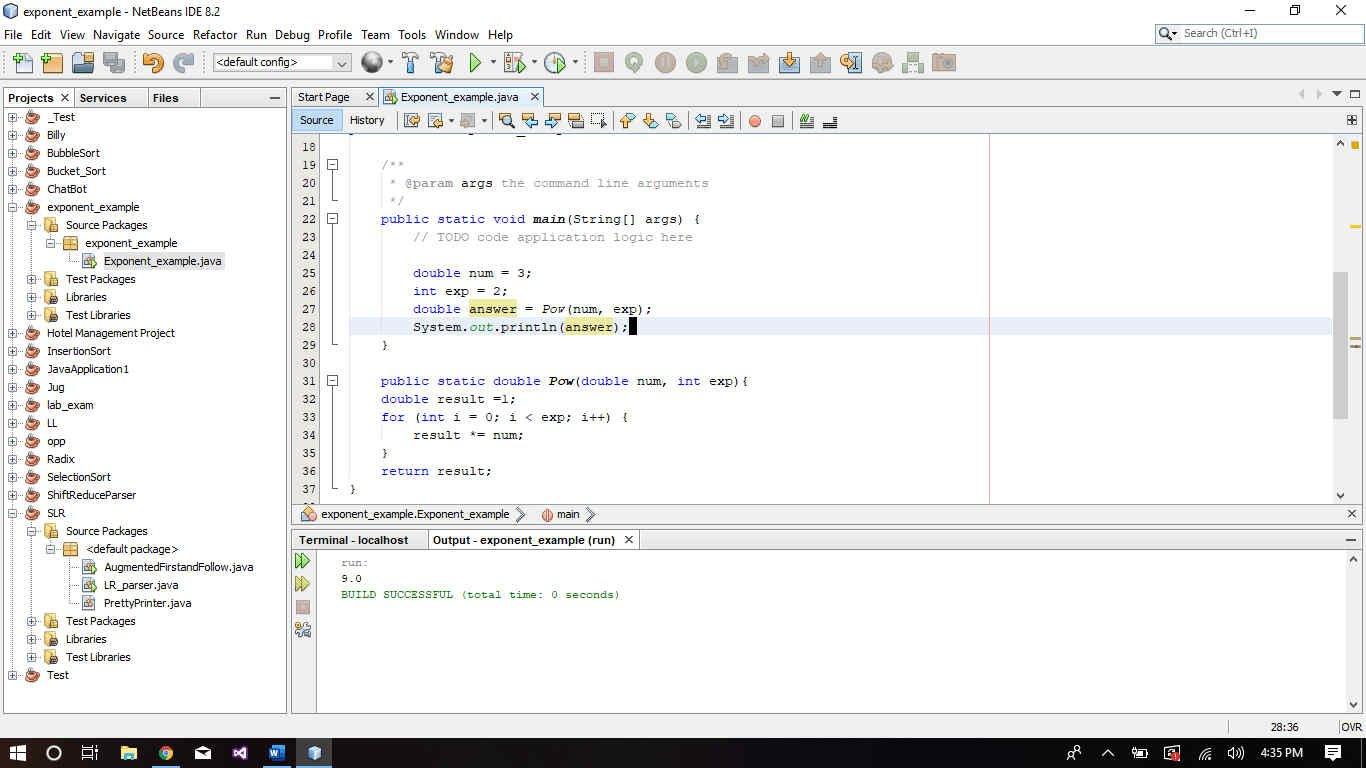## 3. Find Exponent in Jave Using Recursive Call

You can also do it by using a recursive call.

```package exponent_example;
public class Exponent_example {
public static void main(String[] args) {
double num = 3;
int exp = 2;
}

public static double Pow(double num, double exp) {
if (exp <= 0)
return 1;

return  num * Pow(num, exp - 1);
}
}```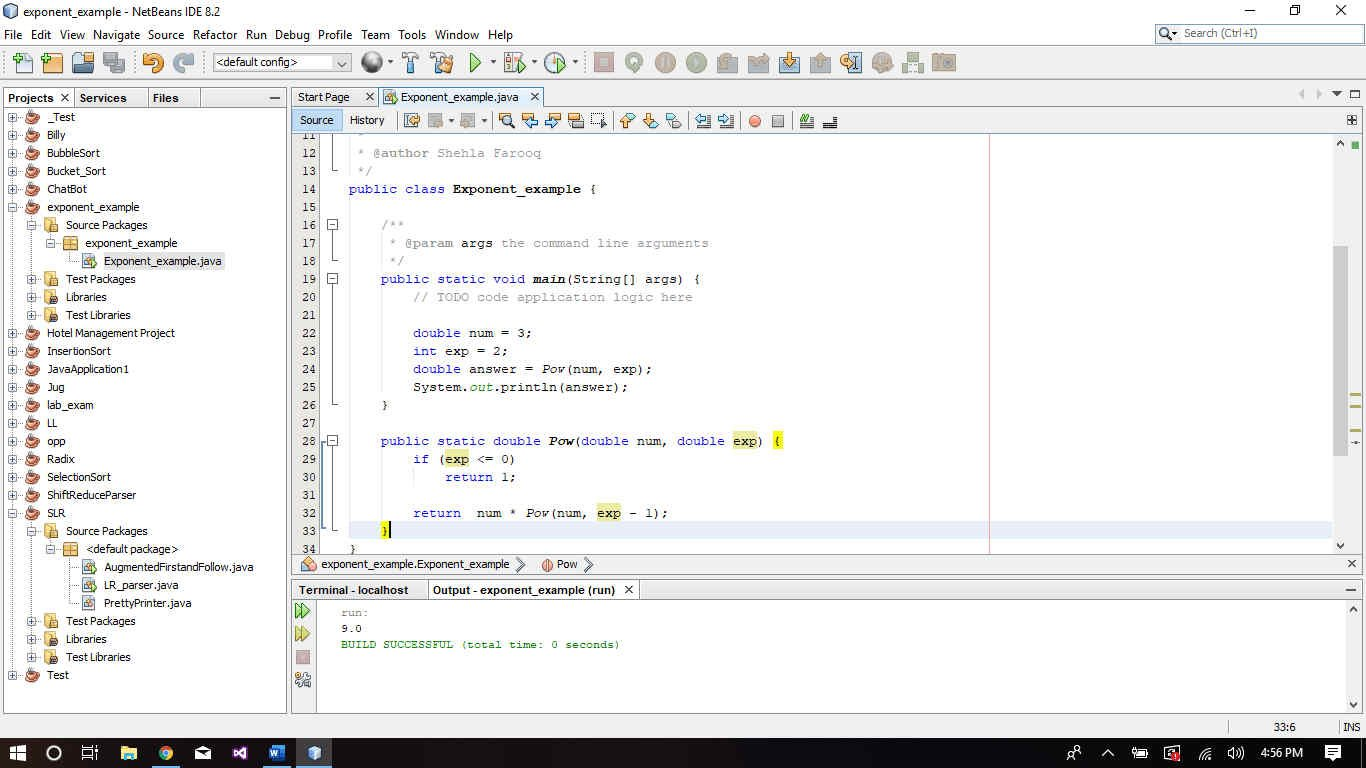You might also like